# DFA 基础简介及简单使用

## DFA 是什么

• 确定：每个状态在一个输入下的转移状态只有一个
• 有限：状态的数量有限

• 一个非空有限状态集合 Set
• 一个输入字母表（非空有限字符集合）Set
• 一个转移函数 (state:State, char:string) => State
• 一个初始状态State
• 一个接受状态集合 Set

1. 类似正则，判断一个字符串是否符合某种规则

eg：考虑以下 ttml 标签，注意 class 的值：

• 合法
``````<view class="primary enable" />

<view class="primary {{ appTheme==='dark'?'dark':'light' }} enable" />

<view class="primary {{ index===1 ? '}}' : '{{' }} enable" />

<view class="primary {{ a + 'b' }} enable" />

• 非法
``````
<view class="primary {{ appTheme==='dark' dark':'light' }} enable" />

<view class="primary {{ appTheme==='dark' 'dark':'light' }} enable" />

1. 解析/序列化一个字符串 eg：CSS 属性 `transition` 语法为
``````div {
transition: <property> <duration> <timing-function> <delay>;
}

### 使用案例1 - 解析 ttml 模板标签属性，即判断一个 ttml 某个标签的某个带模板的属性值是否合法，以及该值属性值的语义

1. 输入字母表

• 识模板部分的 `{` `}`
• 标识字符串部分的 `'` `"`
• 其余所有的字符用 `other` 表示

``````enum ALPHABET {
LEFT_BRACE = 1, // 左括号
RIGHT_BRACE, // 右括号
SINGLE_QUOTE, // 单引号
DOUBLE_QUOTE, // 双引号
OTHER, // 其它字符
}

1. 初始状态

1. 转移函数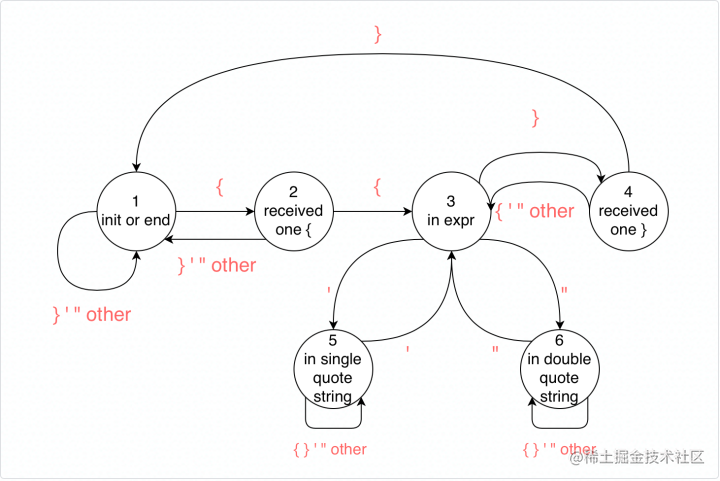``````const MOVE_MATRIX = [ // 状态转移矩阵
[2, 1, 1, 1, 1],
[3, 1, 1, 1, 1],
[3, 4, 5, 6, 3],
[3, 1, 3, 3, 3],
[5, 5, 3, 5, 5],
[6, 6, 6, 3, 6],
];

1. 状态集合 由状态转移图自然可以得到 6 个状态
``````enum STATE { // 状态集合
INIT_OR_END = 1, // 初始 or 终止状态
RECEIVED_ONE_LEFT_BRACE, // 已接受一个 {
IN_EXPR, // 在表达式中，即已接受两个 {
RECEIVED_ONE_RIGHT_BRACE, // 已接受一个 }
IN_EXPR_SINGLE_QUOTE, // 在表达式中的单引号字符串中
IN_EXPR_DOUBLE_QUOTE, // 在表达式中的双引号字符串中
}

1. 接受状态集合 显然只有一个状态可以被接受，即 状态 1：`INIT_OR_END`
``````const FINAL_STATES = new Set([STATE.INIT_OR_END]);

``````enum STATE { // 状态集合
INIT_OR_END = 1,
IN_EXPR,
IN_EXPR_SINGLE_QUOTE,
IN_EXPR_DOUBLE_QUOTE,
}
enum ALPHABET { // 输入字母表
LEFT_BRACE = 1,
RIGHT_BRACE,
SINGLE_QUOTE,
DOUBLE_QUOTE,
OTHER,
}
const MOVE_MATRIX = [
// 状态转移矩阵
[2, 1, 1, 1, 1],
[3, 1, 1, 1, 1],
[3, 4, 5, 6, 3],
[3, 1, 3, 3, 3],
[5, 5, 3, 5, 5],
[6, 6, 6, 3, 6],
];
const move = (state: STATE, char: ALPHABET) => {
// 状态转移函数
return MOVE_MATRIX[state - 1][char - 1]; // 因为状态枚举中，数字是从1而不是从0开始的，所以用于数组索引时要-1
};

const FINAL_STATES = new Set([STATE.INIT_OR_END]); // 接受状态集合，这里就一个状态 1
const INIT_STATE = STATE.INIT_OR_END; // 初始状态 1

function mapCharToAlphabet(char: string): ALPHABET {
// 将每个字符映射到字母表里
switch (char) {
case `{`:
return ALPHABET.LEFT_BRACE;
case `}`:
return ALPHABET.RIGHT_BRACE;
case `'`:
return ALPHABET.SINGLE_QUOTE;
case `"`:
return ALPHABET.DOUBLE_QUOTE;
default:
return ALPHABET.OTHER;
}
}

interface Expr {
type: SLICE_TYPE;
value: string;
}

export enum SLICE_TYPE {
NORMAL = 'normal',
EXPR = 'expr',
}

export default function tokenize(text: string): {
success: boolean;
exprs: Expr[];
} {
let char = ALPHABET.OTHER;
let prevState = INIT_STATE;
let state = INIT_STATE;

let sectionStart = 0;
let sectionEnd = 0;

const exprs: Expr[] = [];

function emit(type: SLICE_TYPE) {
// 往 exprs 里塞一个元素
const slice = text.slice(sectionStart, sectionEnd + 1);
if (slice.length > 0) {
exprs.push({ type, value: slice });
}
}

for (let i = 0; i < text.length; i++) {
char = mapCharToAlphabet(text.charAt(i));
prevState = state;
state = move(state, char); // 状态转移

if (
prevState === STATE.RECEIVED_ONE_LEFT_BRACE &&
state === STATE.IN_EXPR
) {
// 从2转移到3，说明进入了一个 expr
sectionEnd = i - 2;
emit(SLICE_TYPE.NORMAL); // emit 该 expr 之前的部分，一定是 normal text（两个紧挨着的 expr 中间的空字符串会在 emit 中被过滤掉）
sectionStart = i - 1;
} else if (
prevState === STATE.RECEIVED_ONE_RIGHT_BRACE &&
state === STATE.INIT_OR_END
) {
// 从4转移到5，说明退出了一个 expr
sectionEnd = i;
emit(SLICE_TYPE.EXPR); // emit 该 expr
sectionStart = i + 1;
}
}

sectionEnd = text.length - 1;
emit(SLICE_TYPE.NORMAL); // emit 最后一部分 normal text（如果有）

if (FINAL_STATES.has(state)) {
// 如果最终状态在接受状态集合里，说明输入合法，解析成功
return {
success: true,
exprs,
};
} else {
return {
success: false,
exprs: [],
};
}
}

• 合法
``````<view class="primary enable" />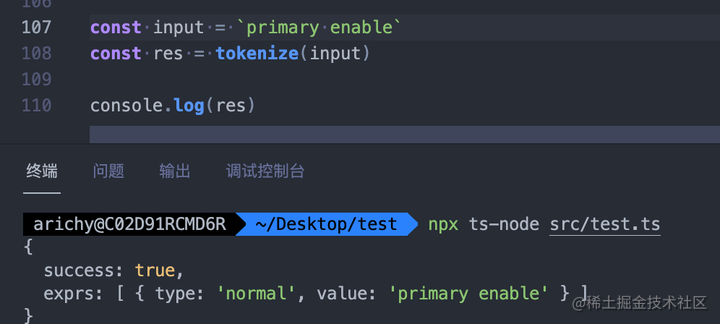``````<view class="primary {{ appTheme==='dark'?'dark':'light' }} enable" />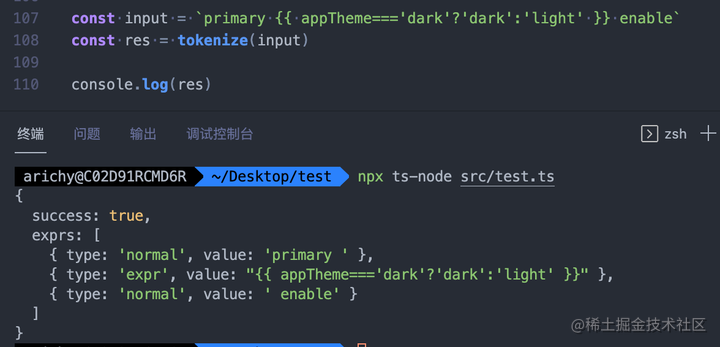``````<view class="primary {{ index===1 ? '}}' : '{{' }} enable" />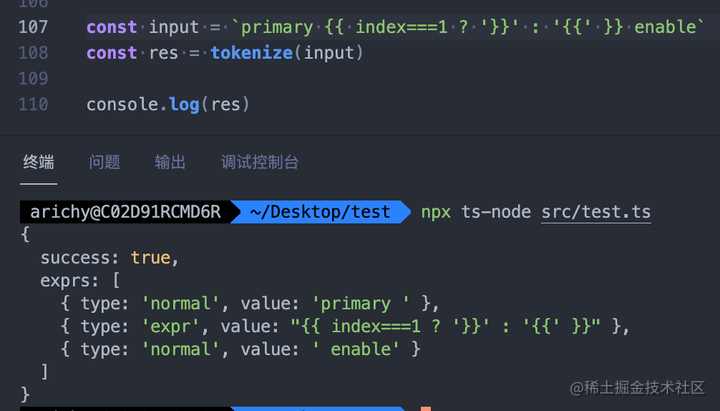``````<view class="primary {{ a + "b" }} enable" />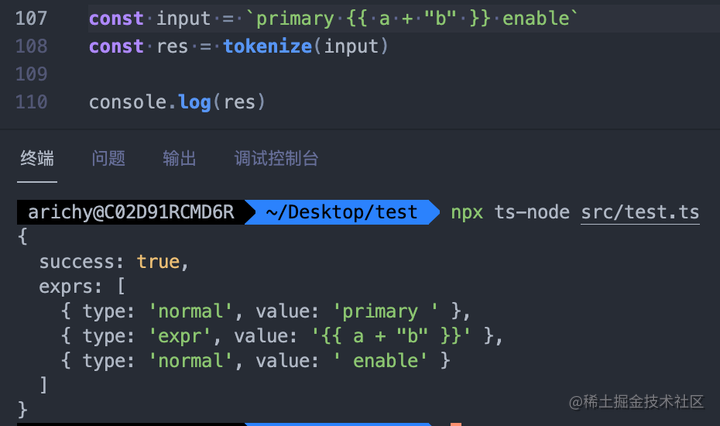• 非法
``````<view class="primary {{ appTheme==='dark' dark':'light' }} enable" />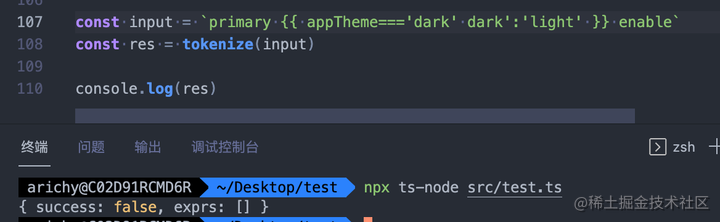ttml 属性值非法。非法原因：模板中引号未完全匹配

``````<view class="primary {{ appTheme==='dark' 'dark':'light' }} enable" />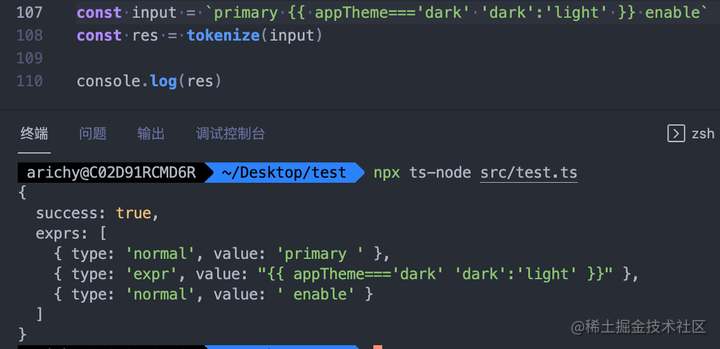ttml 属性值合法，但模板中 JS 非法，此时可将该 JS expression 传给其他 JS 解析器例如 babel-parser 去验证合法性。

### 使用案例2 - 解析 CSS transition 属性，使 JS 可以方便读写该属性

css `transition``<property> <duration> <timing-function> <delay>` 四个值的合并简写。其本身的解析规则为：

• 由 1 - 4 个部分组成（空格隔开），否则非法
• 4 个部分按照字符串类型分为"时间"、"timing-function"、"其他"
• 时间最多出现 2 个，否则非法。第一个作为 `duration` ，第二个作为 `delay`
• 去掉时间部分
• 如果还剩下 1 个部分：该部分若为 timing-function，则作为 `timing-function`。否则作为 `property`
• 如果还剩下 2 个部分 a 和 b：
• a b 均为 timing-function：a 作为 `timing-function,`b 作为 `property`
• a 为 timing-function，b 为其他：a 作为 `timing-function`,b 作为 `property`
• a 为其他，b 为 timing-function：a 作为 `property`，b 作为 `timing-function`
• a b 均为其他：非法
• 如果剩下 > 2 个部分：非法

• 对时间定义
• 第一个非时间部分作为 property，第二个非时间部分作为 timing-function
• 第一个时间部分作为 duration，第二个时间部分作为 delay

1. 输入字母表

• 空格，用于区分每个部分
• 数字，包含 0 - 9 和 `.`
• 左括号 `(`
• 右括号 `)`
• 逗号`,`用于分隔属性
• 其它
1. 初始状态

1. 转移函数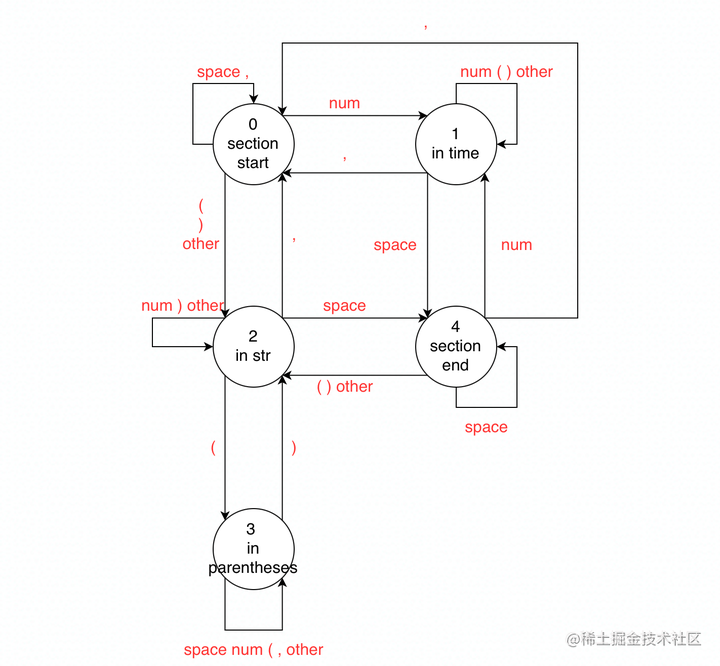``````const MATRIX = [
[0, 1, 2, 2, 0, 2],
[4, 1, 1, 1, 0, 1],
[4, 2, 3, 2, 0, 2],
[3, 3, 3, 2, 3, 3],
[4, 1, 2, 2, 0, 2],
];

1. 状态集合
``````const STATES = {
START: 0,
IN_TIME: 1,
IN_STR: 2,
IN_PARENTHESES: 3,
END: 4,
};

1. 接受状态集合
``````const FINAL_STATES = new Set([STATES.END]);

• 在每次首次到达 `end` 或者 `start` 状态时，说明此时已经解析完了一个 `section`，根据上述语法分析的规则，将对于的属性赋值为当前解析完毕的 `section`
• 在每次首次到达 `start` 状态时，说明此时已经解析完了一个完整的 `transition`，将之前保存属性的对象赋给最终结果，以 `property` 作为 `key`

``````const STATES = {
START: 0,
IN_TIME: 1,
IN_STR: 2,
IN_PARENTHESES: 3,
END: 4,
};

const ALPHABET = {
SPACE: 0,
NUMBER: 1,
LEFT_PARENTHESE: 2,
RIGHT_PARENTHESE: 3,
COMMA: 4,
OTHERS: 5,
};

const MATRIX = [
[0, 1, 2, 2, 0, 2],
[4, 1, 1, 1, 0, 1],
[4, 2, 3, 2, 0, 2],
[3, 3, 3, 2, 3, 3],
[4, 1, 2, 2, 0, 2],
];

const move = (state, char) => MATRIX[state][char];

const FINAL_STATES = new Set([STATES.END]);
const INIT_STATE = STATES.START;

const mapCharToAlphabet = (char) => {
if (char === '(') {
return ALPHABET.LEFT_PARENTHESE;
}

if (char === ')') {
return ALPHABET.RIGHT_PARENTHESE;
}

if (char === ' ') {
return ALPHABET.SPACE;
}

if (char === ',') {
return ALPHABET.COMMA;
}

if ((char >= '0' && char <= '9') || char === '.') {
return ALPHABET.NUMBER;
}

return ALPHABET.OTHERS;
};

export const parseTransition = (transition) => {
let map = {};

if (transition[transition.length - 1] !== ',') {
transition += ',';
}

let prevState = INIT_STATE;
let state = INIT_STATE;

let sectionStart = 0;
let sectionEnd = 0;

let property = '';
let duration = '';
let timingFunction = '';
let delay = '';

const emitProperty = () => {
let str = transition.slice(sectionStart, sectionEnd);
if (prevState === STATES.IN_STR) {
if (property.length === 0) {
property = str;
} else {
timingFunction = str;
}
} else if (prevState === STATES.IN_TIME) {
if (duration.length === 0) {
duration = str;
} else {
delay = str;
}
}
};

const emit = () => {
if (property.length === 0) {
property = 'all';
}

map[property] = {
property,
duration,
timingFunction,
delay,
};
property = '';
duration = '';
timingFunction = '';
delay = '';
};

let char;

for (let i = 0; i < transition.length; i++) {
char = mapCharToAlphabet(transition[i]);
prevState = state;
state = move(state, char);

if (
(state === STATES.IN_STR || state === STATES.IN_TIME) &&
prevState !== state &&
prevState !== STATES.IN_PARENTHESES
) {
sectionStart = i;
} else if (state === STATES.END && prevState !== state) {
sectionEnd = i;

emitProperty();
} else if (state === STATES.START && prevState !== state) {
sectionEnd = i;

emitProperty();
emit();
}
}

return map;
};

export const stringifyTransition = (map) => {
return Object.values(map)
.map(
({ property, duration, timingFunction, delay }) =>
`\${property} \${duration} \${timingFunction} \${delay}`
)
.join(',');
};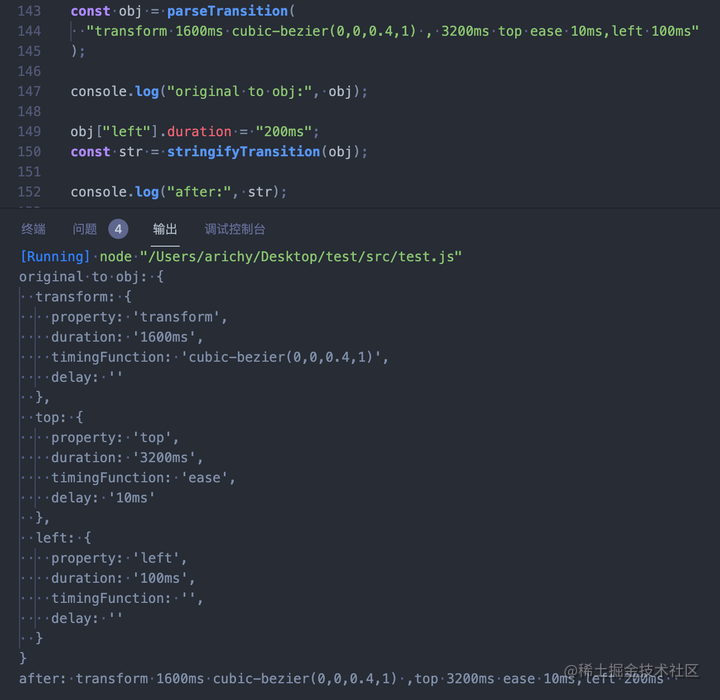MDN - transition